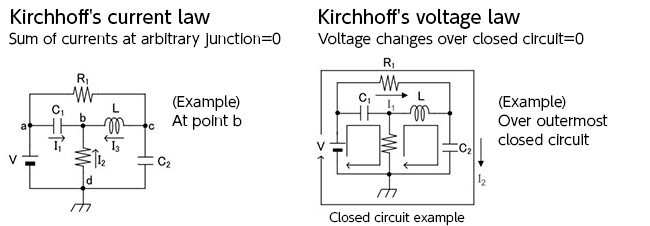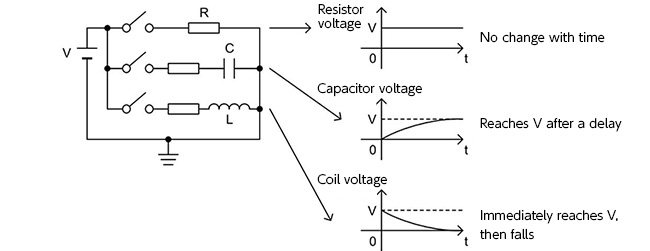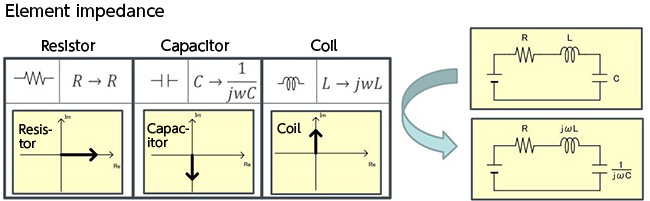Technical Information Site of Power Supply Design

2016.10.20 Transfer Function

# What are transfer functions? - Kirchhoff’s Laws and Impedance

DC/DC Converters: Sharing of Transfer Functions among Control Systems

Before actually determining transfer functions block-by-block, two important laws for deriving transfer functions are first checked.

One is Kirchhoff's current law. This is a law that states that "at an arbitrary junction, the sum of the currents is zero". In considering this law, it is vital to pay attention to the directions of the currents.

The other is Kirchhoff's voltage law. This law states that "over an arbitrary closed circuit, the voltage change is zero." These two laws can be illustrated as in Fig. 3Fig. 3

The above two laws are used in order to derive a transfer function, but there is one other matter that must be studied. That is the matter of how to represent impedance. As indicated in Fig. 4 below, when a DC power supply V is connected to a resistor R, and a capacitor C and a coil L, each behaves differently. The voltage across the resistor R does not change even as time passes. The voltage across the capacitor gradually rises, finally reaching the voltage of the power supply after a certain time has elapsed. The voltage across the coil immediately reaches the voltage of the power supply, but then gradually declines.Fig. 4

From the characteristics shown in Fig. 4, when considering capacitors and coils as resistances, the resistance value (impedance) must be considered as a function of time (phase). This, including resistance that changes with time in this way, is called impedance notation. We say that the impedance of a capacitor rises as time passes as the step response to an input voltage. The behavior of a coil is the opposite of this. In an electrical circuit, an angular velocity ω which is the reciprocal of the time is used, and so the impedance is represented as in Fig. 5.Fig. 5

Finally, the reason for the appearance of complex numbers is explained. In electrical circuits, complex numbers are used to represent phases, as parameters related to response times. In this case, they represent the response times of power supplies. In the case of capacitors, there is a lag before reaching the power supply voltage V; in the case of coils, it is the opposite. This situation can be represented as in Fig. 5.

This website uses cookies.

By continuing to browse this website without changing your web-browser cookie settings, you are agreeing to our use of cookies.